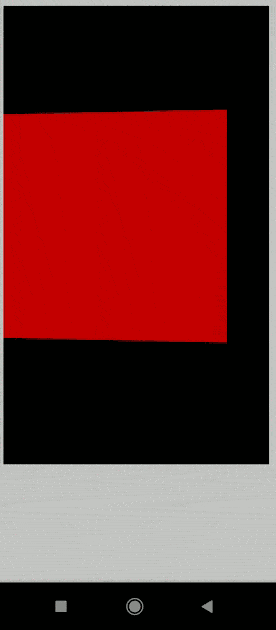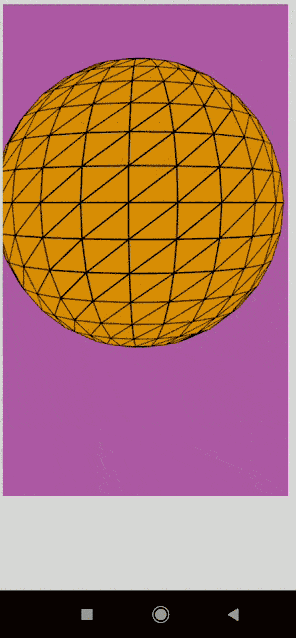# p5.js rotationY Event

• Last Updated : 25 Jul, 2022

The system variable rotationY in p5.js is responsible for the rotation of mobile devices (smartphones and tablets) always along the y-axis. It can be used in the draw() function to continuously get the current rotation along the y-axis. If the graphics angleMode() is set to DEGREES, then the value will be in the range of -90 to 90. When it is set to RADIANS, the value will be between -PI/2 to PI/2.

Note: The order the rotations are called is important if all the three variables are used together. It is required to call them in the order Z, Y, and X to prevent inconsistencies.

Syntax:

`rotationY`

Example 1:

## Javascript

 `// Rotate the device at 90 degree.` `function` `setup() {` `  ``createCanvas(600, 600, WEBGL);` `}`   `function` `draw() {` `  ``background(2);` `  `  `  ``// Set the rotation to be equal to` `  ``// the variable rotationY` `  ``rotateY(radians(rotationY));` `  `  `  ``fill(``'red'``);` `  ``box(200, 200, 200);` `}`

Output:Example 2:

## Javascript

 `// Rotate the device at  0 to 360 degree.` `function` `setup() {` `  ``createCanvas(600, 600, WEBGL);` `}`   `function` `draw() {` `  ``background(205, 105, 194);` `  `  `  ``// Set the rotation to be equal to` `  ``// the variable rotationY` `  ``rotateY(radians(rotationY));` `  `  `  ``fill(``'orange'``);` `  ``sphere(140);` `}`

Output:My Personal Notes arrow_drop_up
Recommended Articles
Page :